# Probabilistic Robotics Probabilistic Motion Models Robot Motion Robot

• Slides: 32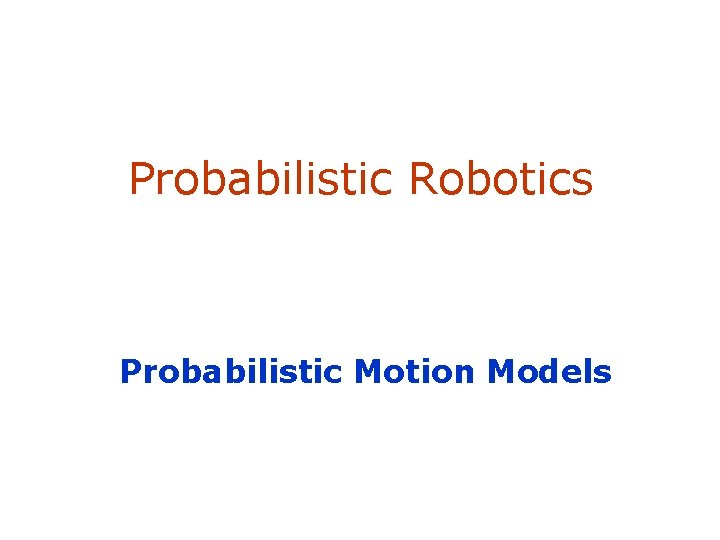Probabilistic Robotics Probabilistic Motion Models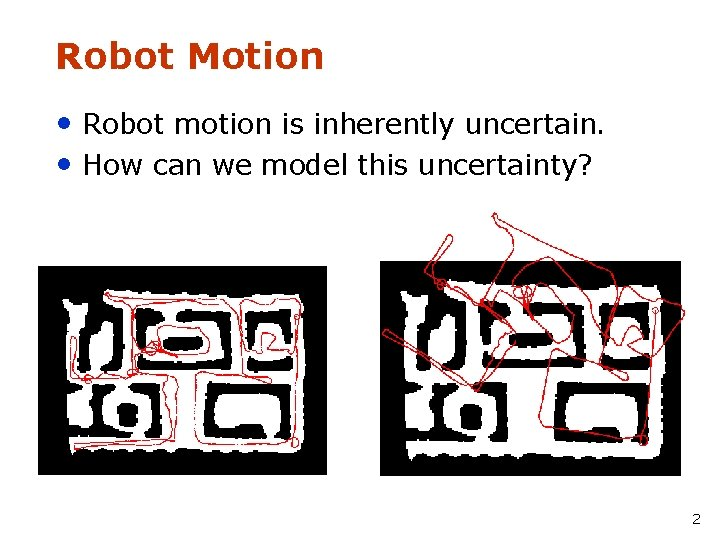Robot Motion • Robot motion is inherently uncertain. • How can we model this uncertainty? 2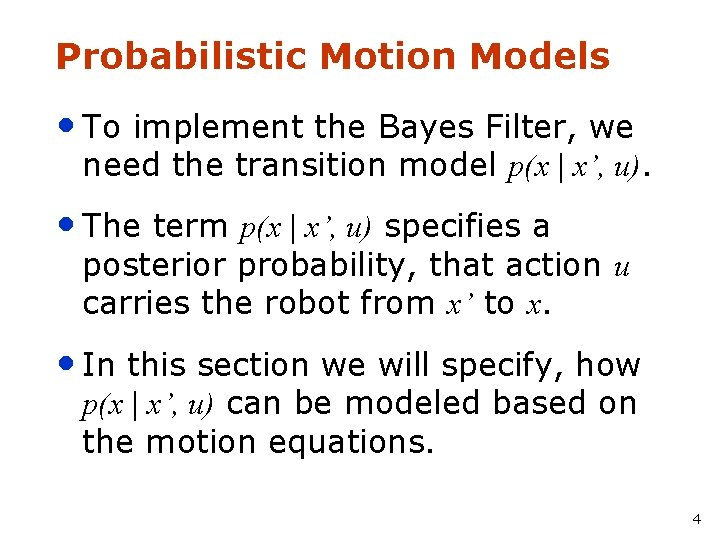Probabilistic Motion Models • To implement the Bayes Filter, we need the transition model p(x | x’, u). • The term p(x | x’, u) specifies a posterior probability, that action u carries the robot from x’ to x. • In this section we will specify, how p(x | x’, u) can be modeled based on the motion equations. 4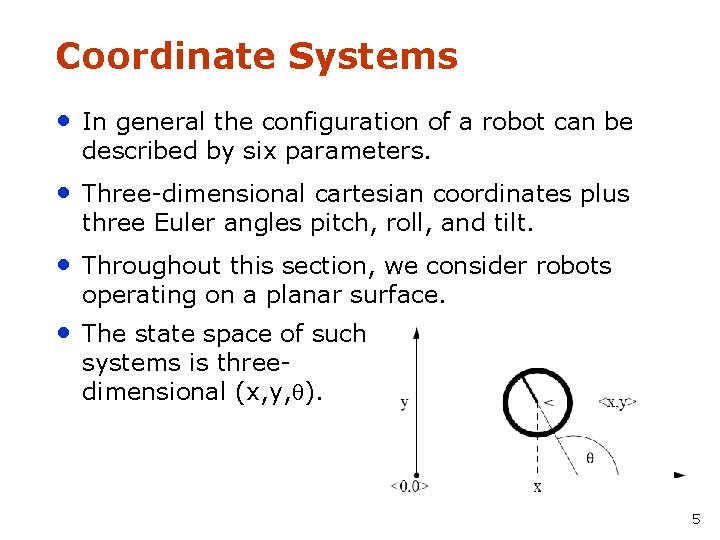Coordinate Systems • In general the configuration of a robot can be described by six parameters. • Three-dimensional cartesian coordinates plus three Euler angles pitch, roll, and tilt. • Throughout this section, we consider robots operating on a planar surface. • The state space of such systems is threedimensional (x, y, ). 5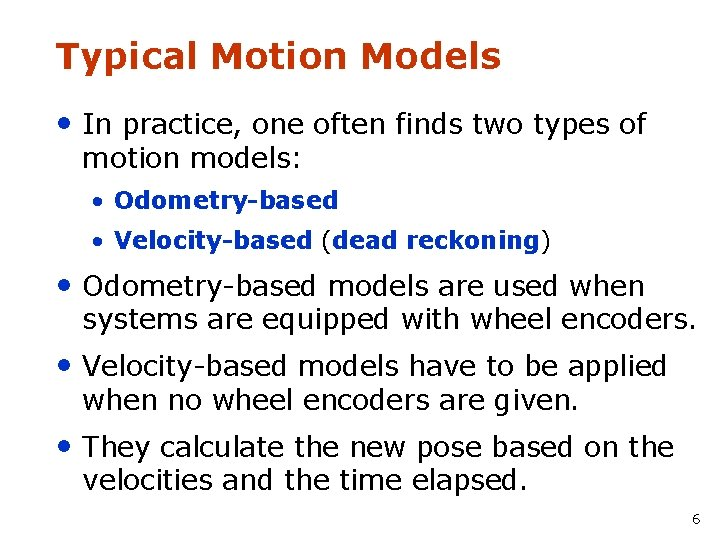Typical Motion Models • In practice, one often finds two types of motion models: • Odometry-based • Velocity-based (dead reckoning) • Odometry-based models are used when systems are equipped with wheel encoders. • Velocity-based models have to be applied when no wheel encoders are given. • They calculate the new pose based on the velocities and the time elapsed. 6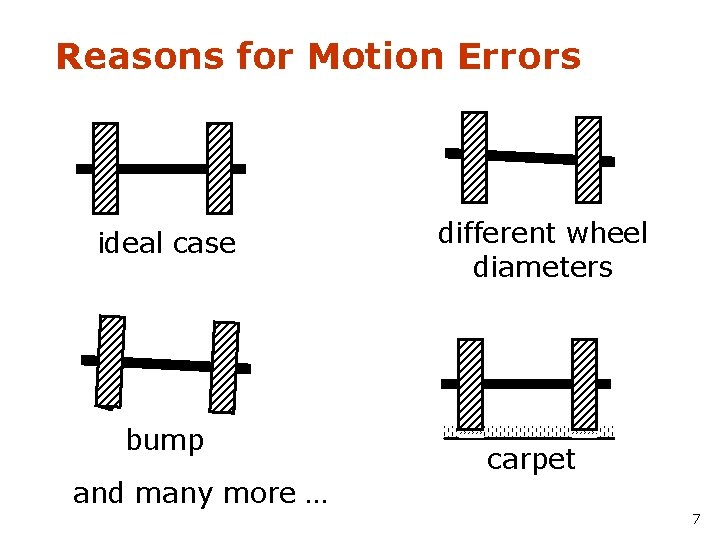Reasons for Motion Errors ideal case bump different wheel diameters carpet and many more … 7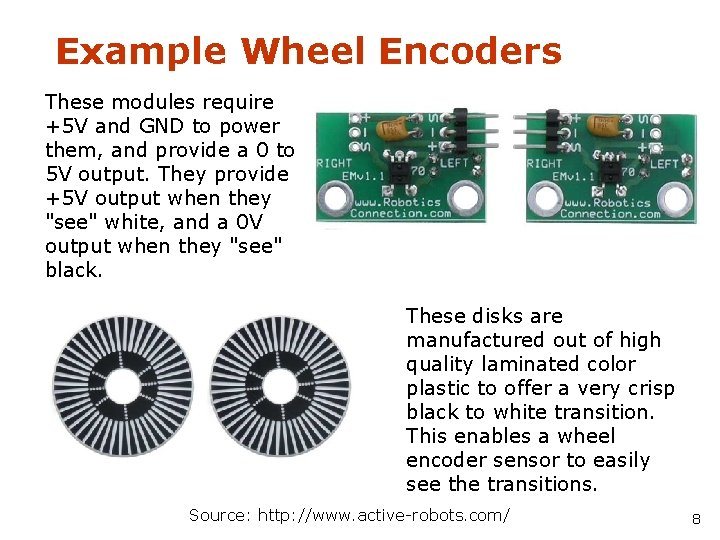Example Wheel Encoders These modules require +5 V and GND to power them, and provide a 0 to 5 V output. They provide +5 V output when they "see" white, and a 0 V output when they "see" black. These disks are manufactured out of high quality laminated color plastic to offer a very crisp black to white transition. This enables a wheel encoder sensor to easily see the transitions. Source: http: //www. active-robots. com/ 8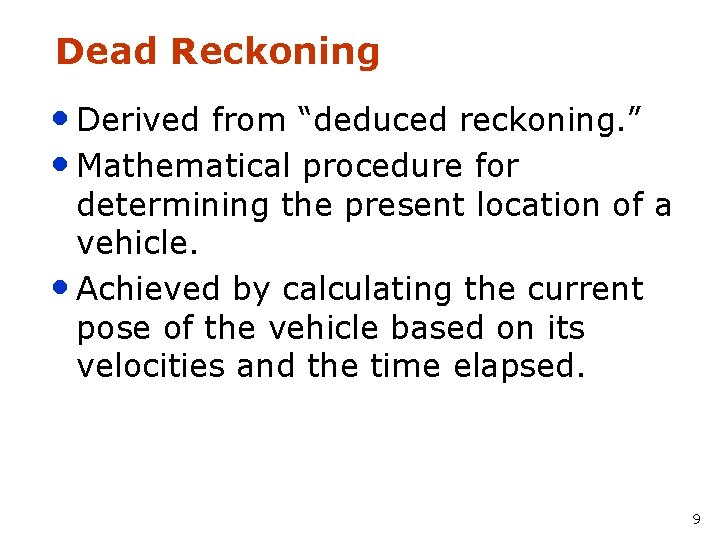Dead Reckoning • Derived from “deduced reckoning. ” • Mathematical procedure for determining the present location of a vehicle. • Achieved by calculating the current pose of the vehicle based on its velocities and the time elapsed. 9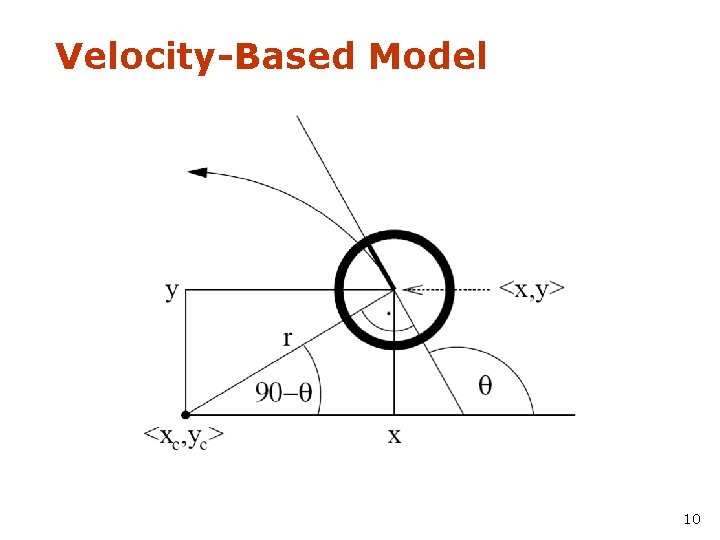Velocity-Based Model 10Equation for the Velocity Model Center of circle: with 11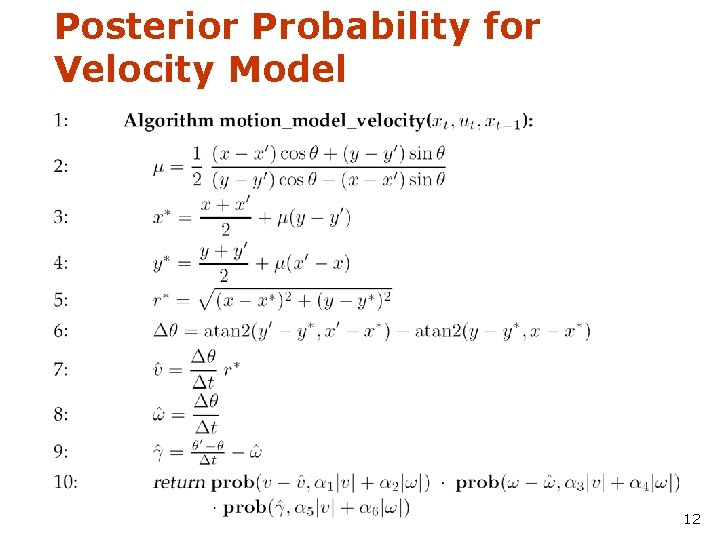Posterior Probability for Velocity Model 12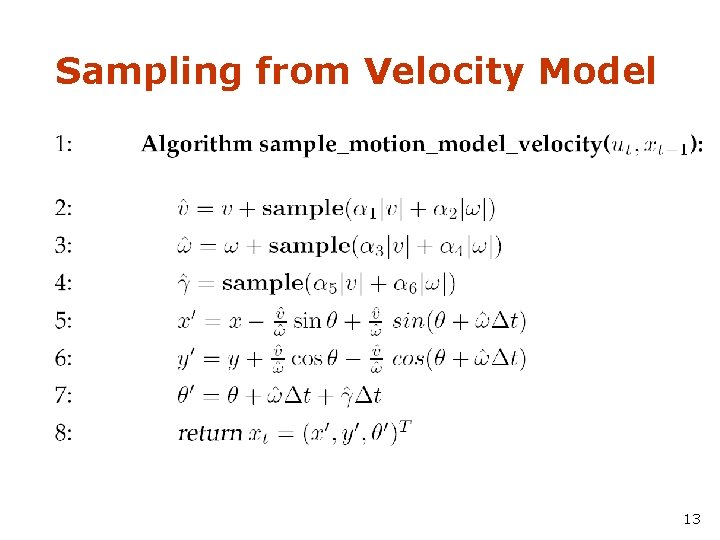Sampling from Velocity Model 13Examples (velocity based) 14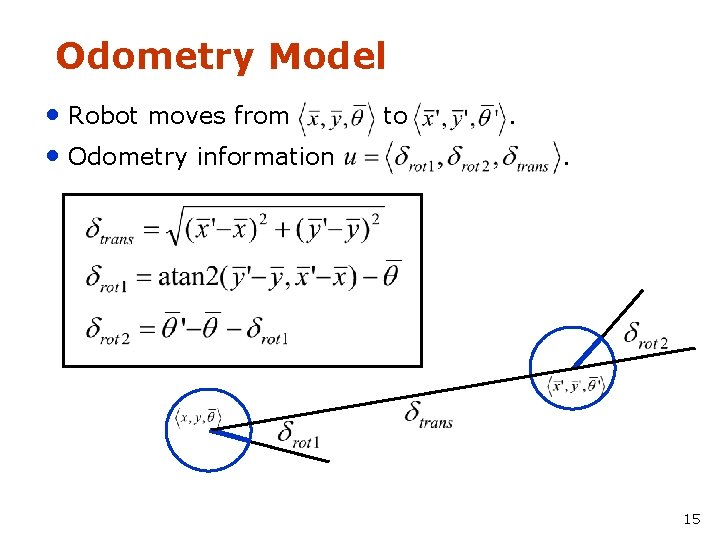Odometry Model • Robot moves from • Odometry information to . . 15The atan 2 Function • Extends the inverse tangent and correctly copes with the signs of x and y. 16Noise Model for Odometry • The measured motion is given by the true motion corrupted with noise. 17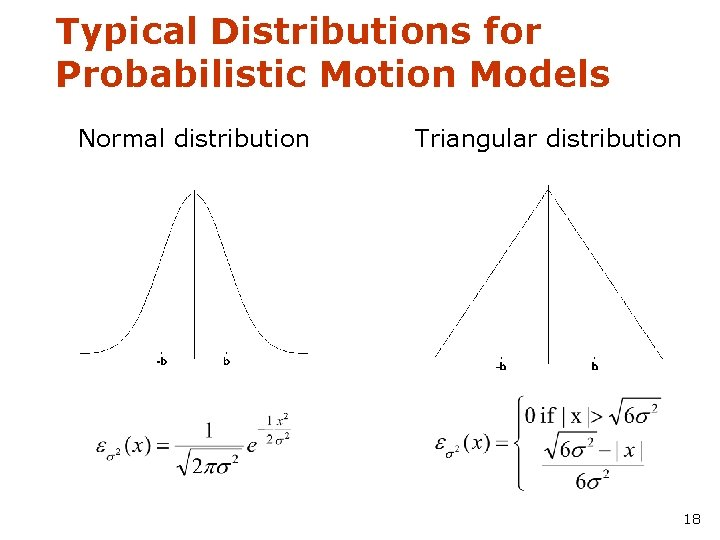Typical Distributions for Probabilistic Motion Models Normal distribution Triangular distribution 18Calculating the Probability (zero-centered) • For a normal distribution 1. Algorithm prob_normal_distribution(a, b): 2. return • For a triangular distribution 1. Algorithm prob_triangular_distribution(a, b): 2. return 19Calculating the Posterior Given x, x’, and u 1. Algorithm motion_model_odometry(x, x’, u) 2. 3. odometry values (u) 4. 5. 6. values of interest (x, x’) 7. 8. 9. 10. 11. return p 1 · p 2 · p 3 20Application • Repeated application of the sensor model • for short movements. Typical banana-shaped distributions obtained for 2 d-projection of 3 d posterior. p(x|u, x’) x’ u 21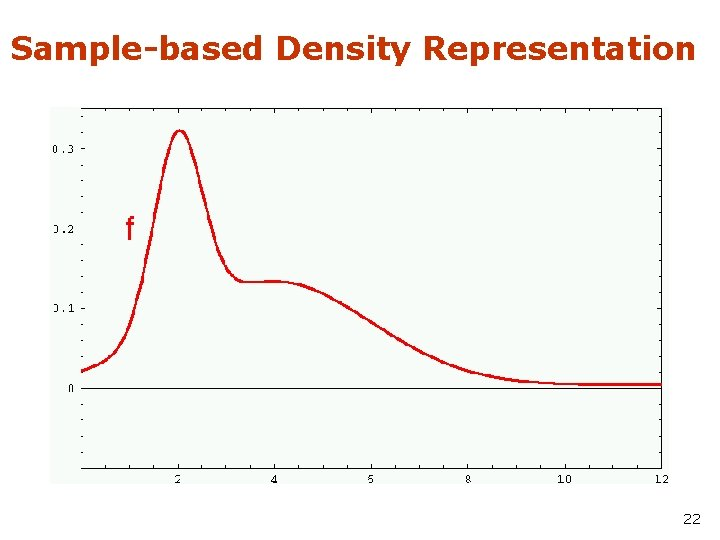Sample-based Density Representation 22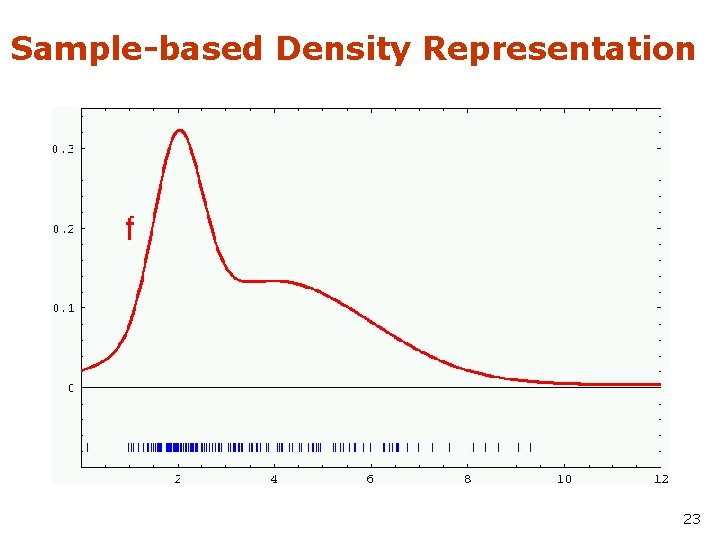Sample-based Density Representation 23How to Sample from Normal or Triangular Distributions? • Sampling from a normal distribution 1. Algorithm sample_normal_distribution(b): 2. return • Sampling from a triangular distribution 1. Algorithm sample_triangular_distribution(b): 2. return 24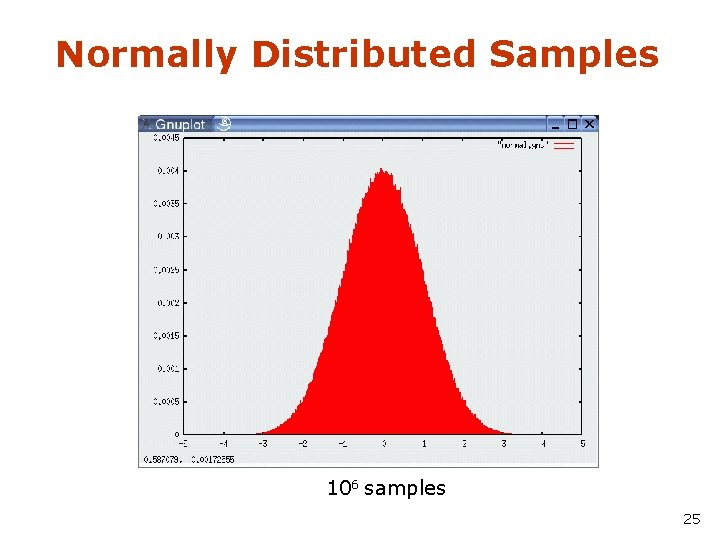Normally Distributed Samples 106 samples 25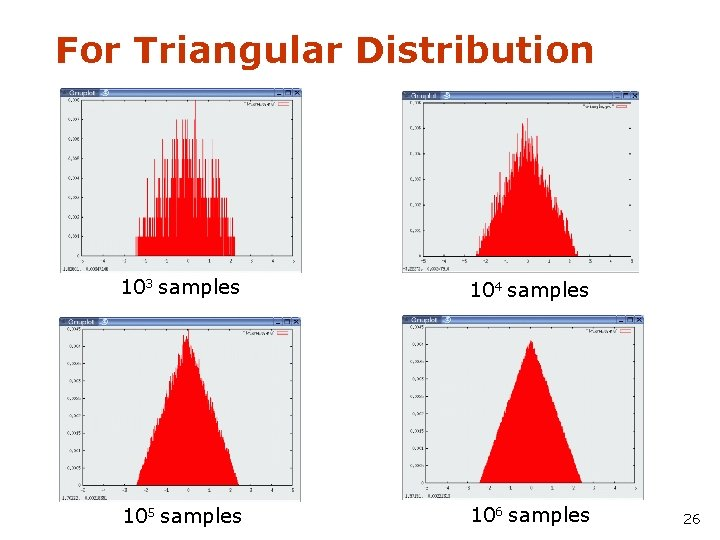For Triangular Distribution 103 samples 104 samples 105 samples 106 samples 26Rejection Sampling • Sampling from arbitrary distributions 1. Algorithm sample_distribution(f, b): 2. repeat 3. 4. 5. until ( 6. return ) 27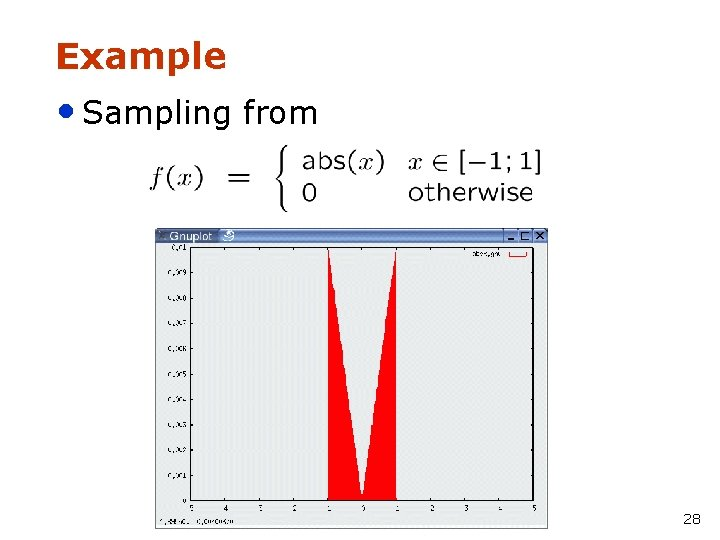Example • Sampling from 28Sample Odometry Motion Model 1. Algorithm sample_motion_model(u, x): 2. 3. 4. 5. 6. 7. sample_normal_distribution 8. 9. Return 29Sampling from Our Motion Model Start 30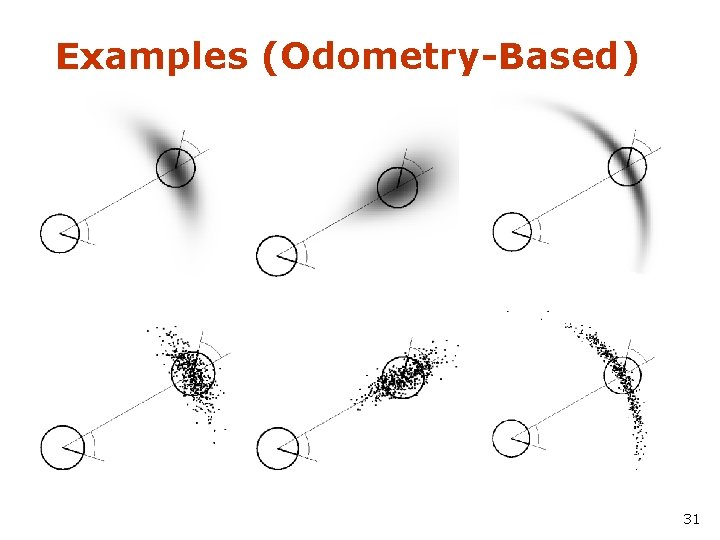Examples (Odometry-Based) 31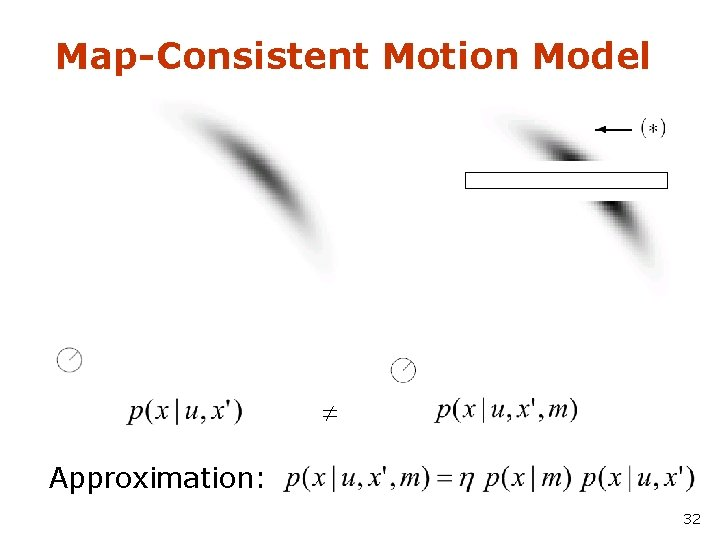Map-Consistent Motion Model Approximation: 32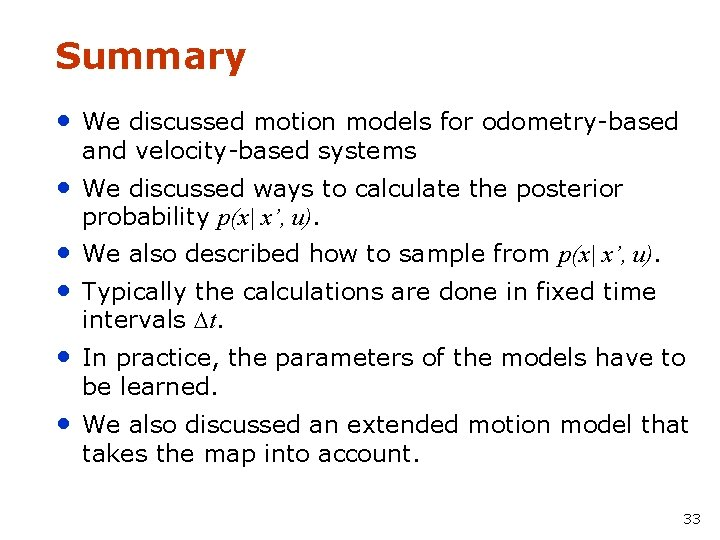Summary • We discussed motion models for odometry-based and velocity-based systems • We discussed ways to calculate the posterior probability p(x| x’, u). • We also described how to sample from p(x| x’, u). • Typically the calculations are done in fixed time intervals t. • In practice, the parameters of the models have to be learned. • We also discussed an extended motion model that takes the map into account. 33